Скачать презентацию Chapter 9 The Cost of Capital The

078b79bcfd5a8e4d5e4f687e48bfa294.ppt

• Количество слайдов: 29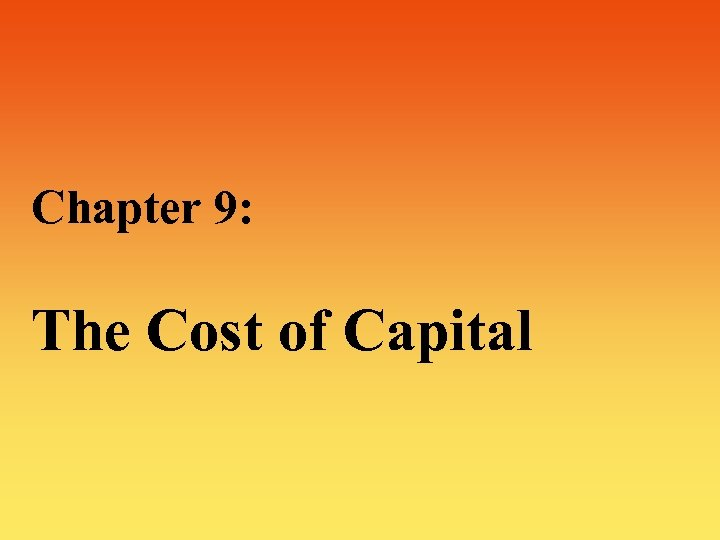Chapter 9: The Cost of Capital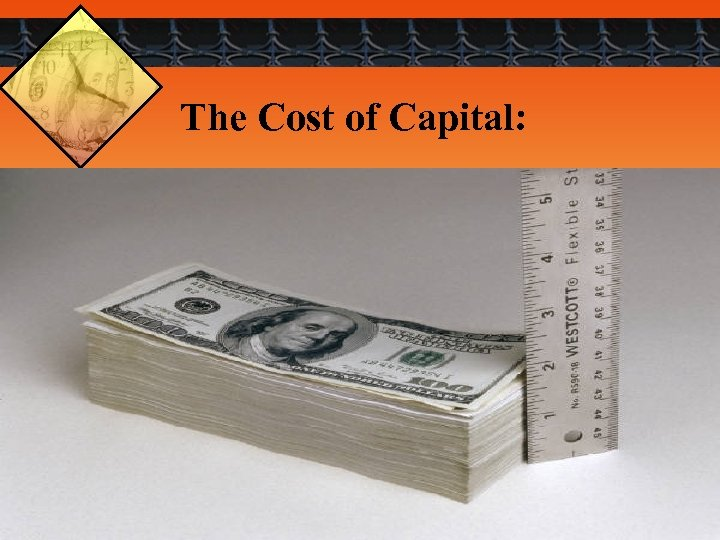The Cost of Capital: 2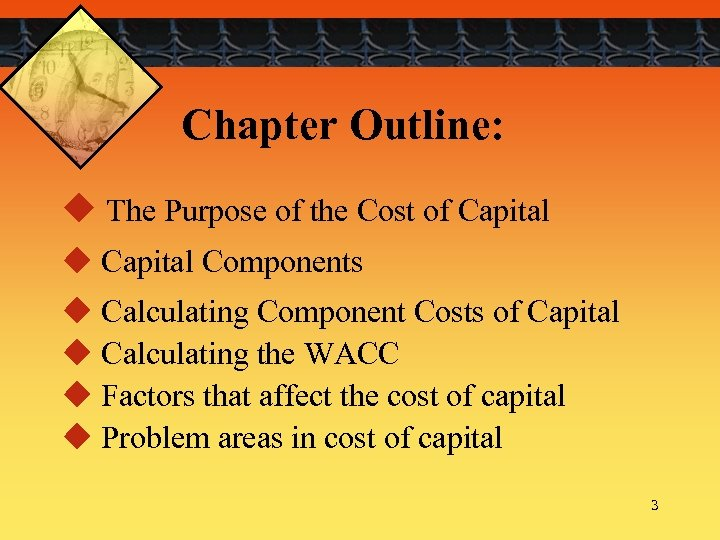Chapter Outline: u The Purpose of the Cost of Capital u Capital Components u Calculating Component Costs of Capital u Calculating the WACC u Factors that affect the cost of capital u Problem areas in cost of capital 3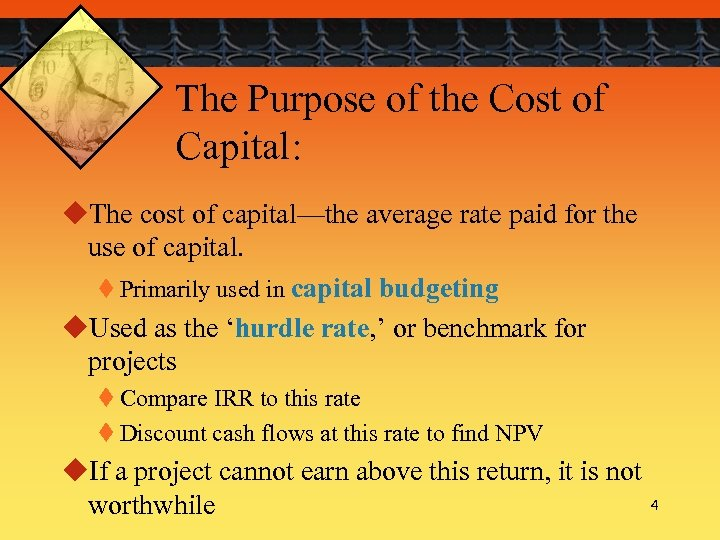The Purpose of the Cost of Capital: u. The cost of capital—the average rate paid for the use of capital. t Primarily used in capital budgeting u. Used as the ‘hurdle rate, ’ or benchmark for projects t Compare IRR to this rate t Discount cash flows at this rate to find NPV u. If a project cannot earn above this return, it is not worthwhile 4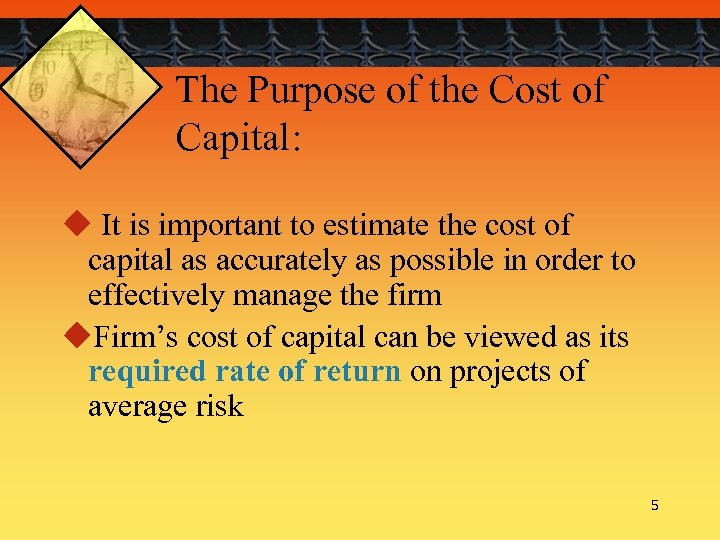The Purpose of the Cost of Capital: u It is important to estimate the cost of capital as accurately as possible in order to effectively manage the firm u. Firm’s cost of capital can be viewed as its required rate of return on projects of average risk 5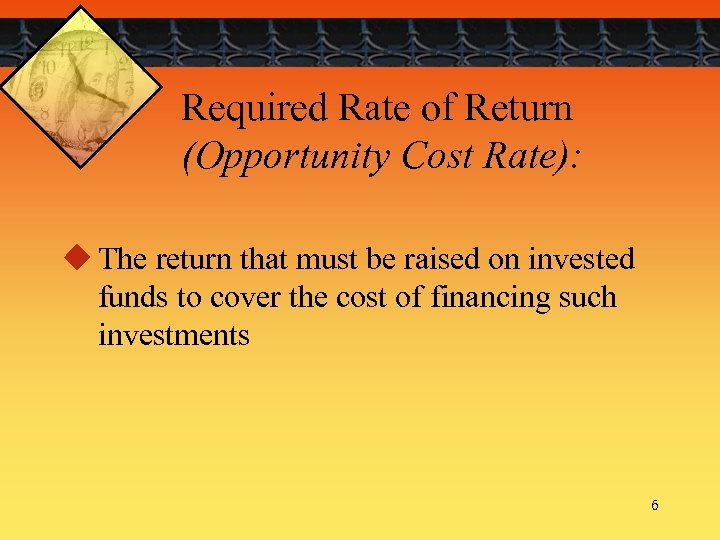Required Rate of Return (Opportunity Cost Rate): u The return that must be raised on invested funds to cover the cost of financing such investments 6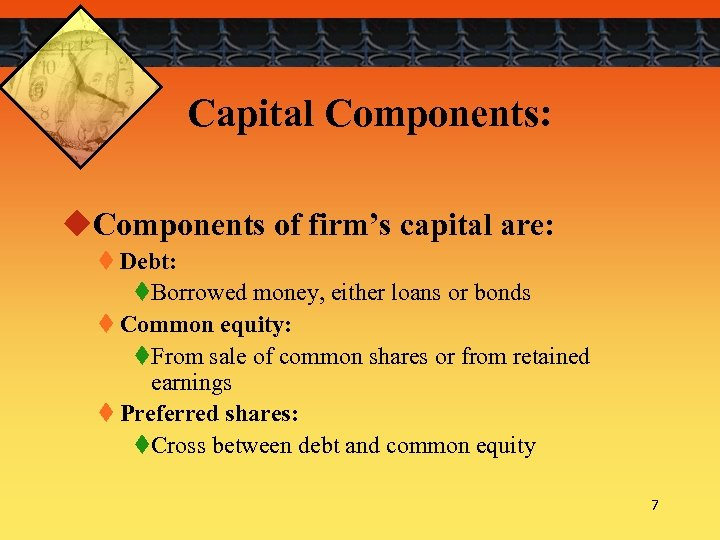Capital Components: u. Components of firm’s capital are: t Debt: t. Borrowed money, either loans or bonds t Common equity: t. From sale of common shares or from retained earnings t Preferred shares: t. Cross between debt and common equity 7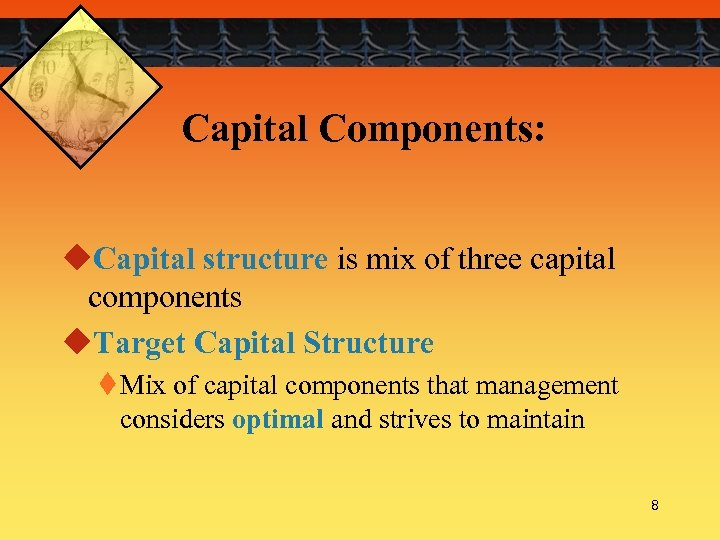Capital Components: u. Capital structure is mix of three capital components u. Target Capital Structure t Mix of capital components that management considers optimal and strives to maintain 8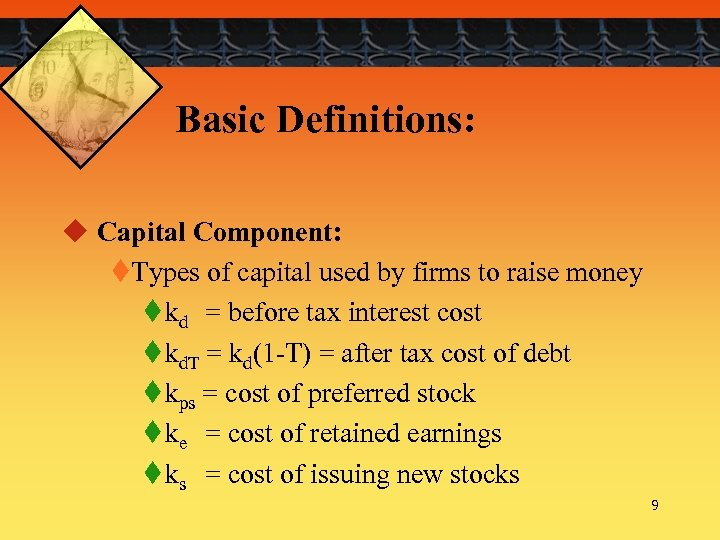Basic Definitions: u Capital Component: t. Types of capital used by firms to raise money t kd = before tax interest cost t kd. T = kd(1 -T) = after tax cost of debt t kps = cost of preferred stock t ke = cost of retained earnings t ks = cost of issuing new stocks 9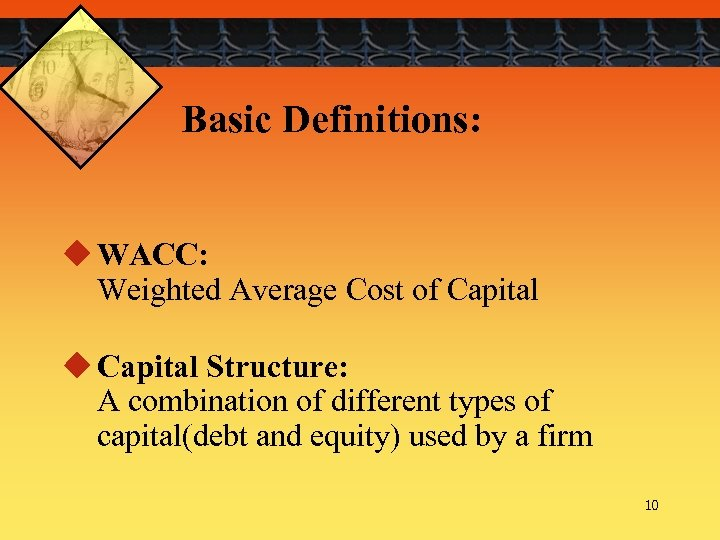Basic Definitions: u WACC: Weighted Average Cost of Capital u Capital Structure: A combination of different types of capital(debt and equity) used by a firm 10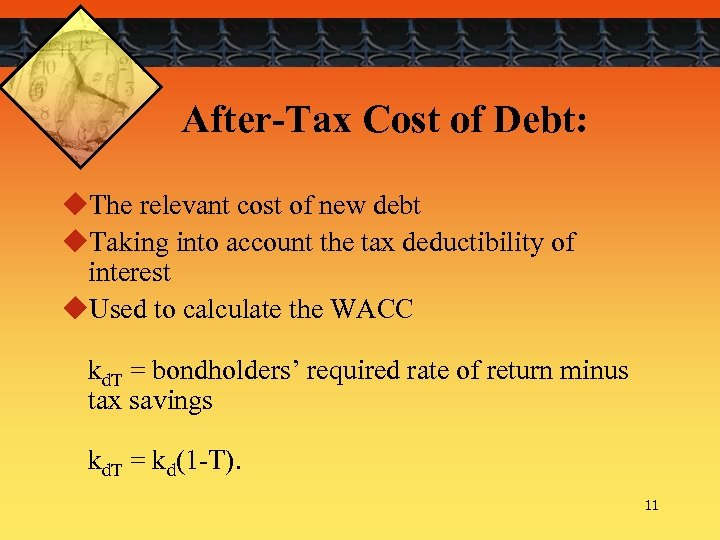After-Tax Cost of Debt: u. The relevant cost of new debt u. Taking into account the tax deductibility of interest u. Used to calculate the WACC kd. T = bondholders’ required rate of return minus tax savings kd. T = kd(1 -T). 11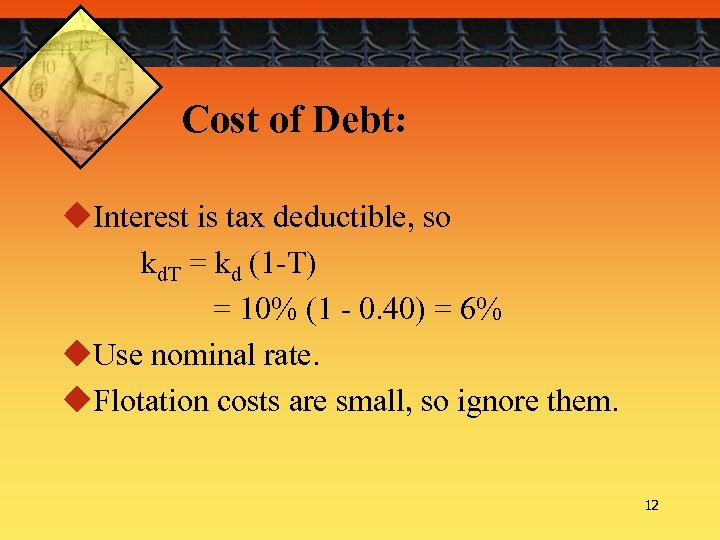Cost of Debt: u. Interest is tax deductible, so kd. T = kd (1 -T) = 10% (1 - 0. 40) = 6% u. Use nominal rate. u. Flotation costs are small, so ignore them. 12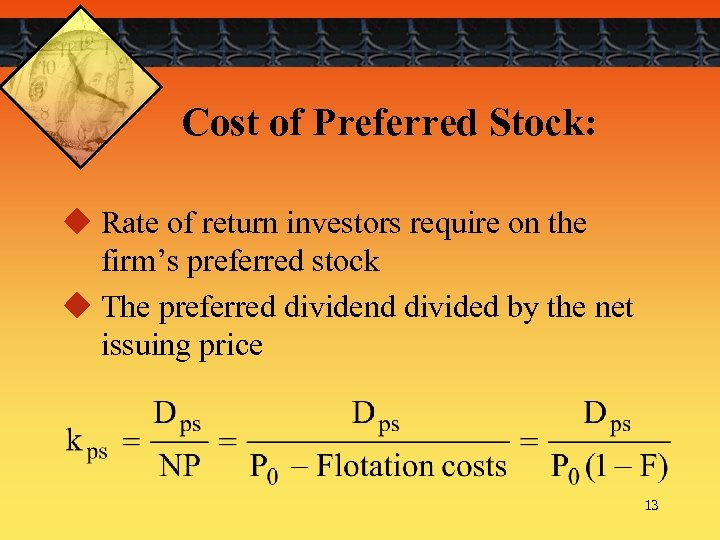Cost of Preferred Stock: u Rate of return investors require on the firm’s preferred stock u The preferred dividend divided by the net issuing price 13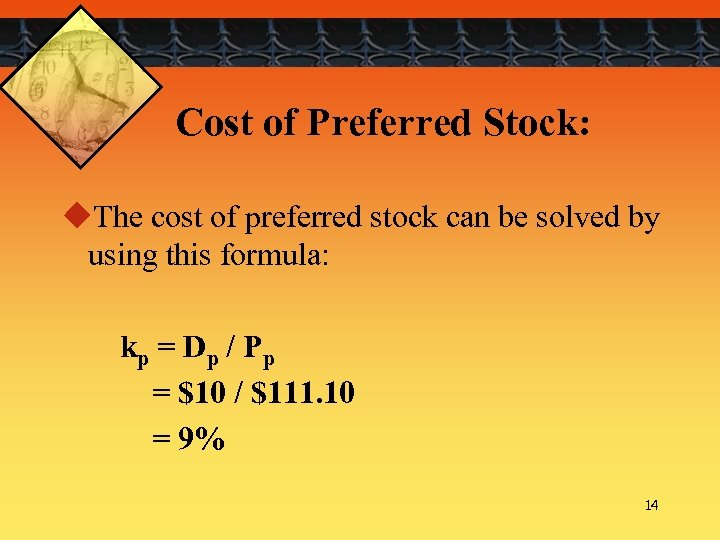Cost of Preferred Stock: u. The cost of preferred stock can be solved by using this formula: kp = D p / P p = \$10 / \$111. 10 = 9% 14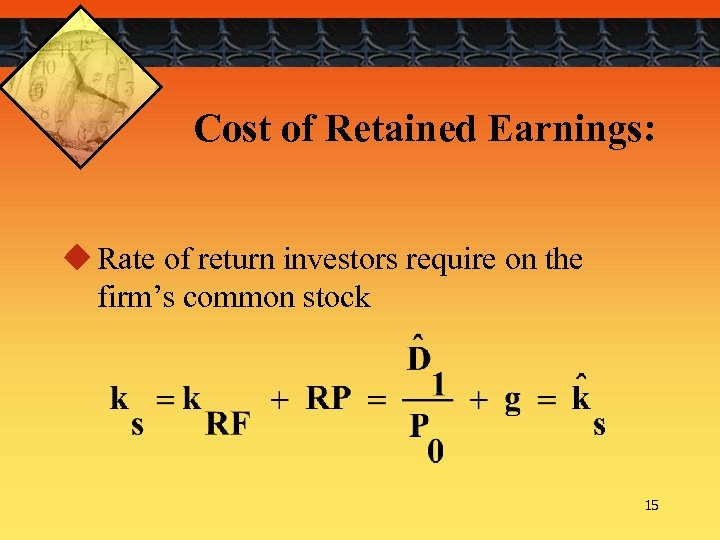Cost of Retained Earnings: u Rate of return investors require on the firm’s common stock 15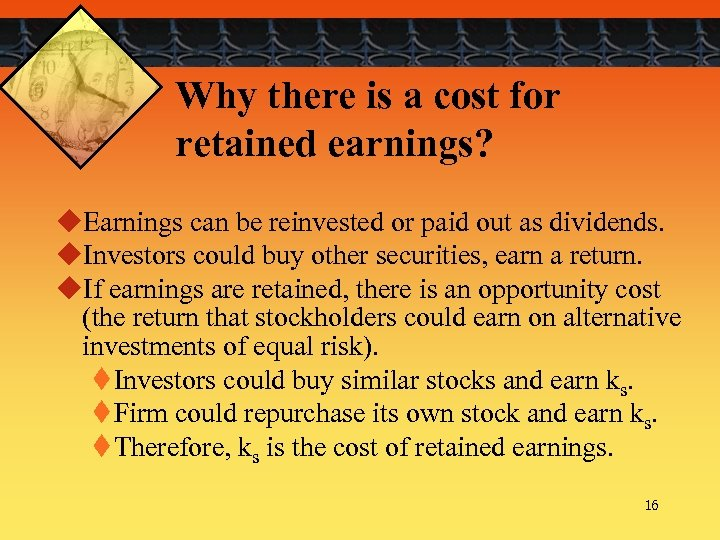Why there is a cost for retained earnings? u. Earnings can be reinvested or paid out as dividends. u. Investors could buy other securities, earn a return. u. If earnings are retained, there is an opportunity cost (the return that stockholders could earn on alternative investments of equal risk). t Investors could buy similar stocks and earn ks. t Firm could repurchase its own stock and earn ks. t Therefore, ks is the cost of retained earnings. 16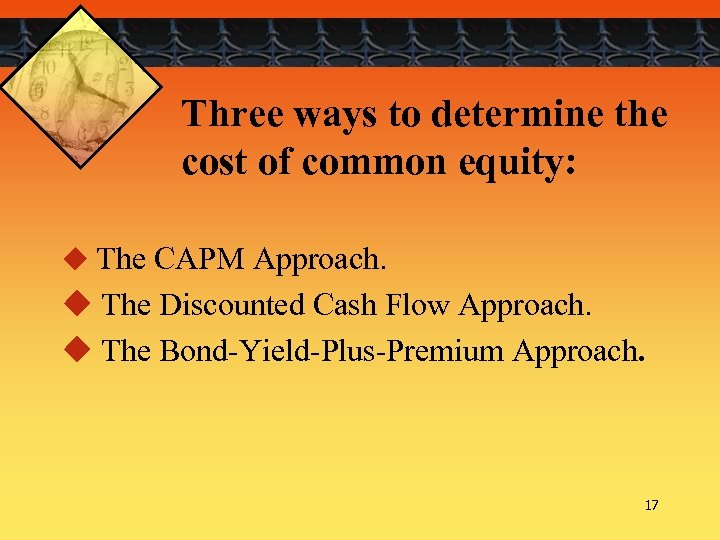Three ways to determine the cost of common equity: u The CAPM Approach. u The Discounted Cash Flow Approach. u The Bond-Yield-Plus-Premium Approach. 17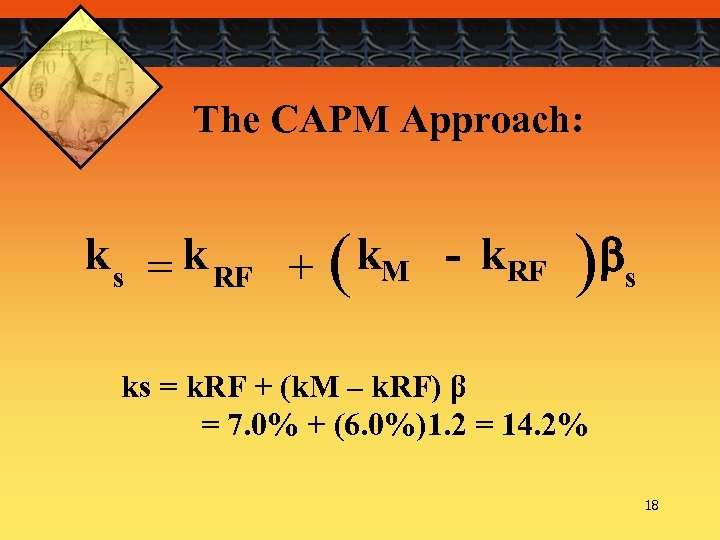The CAPM Approach: k s = k RF + ( k. M - k. RF )bs ks = k. RF + (k. M – k. RF) β = 7. 0% + (6. 0%)1. 2 = 14. 2% 18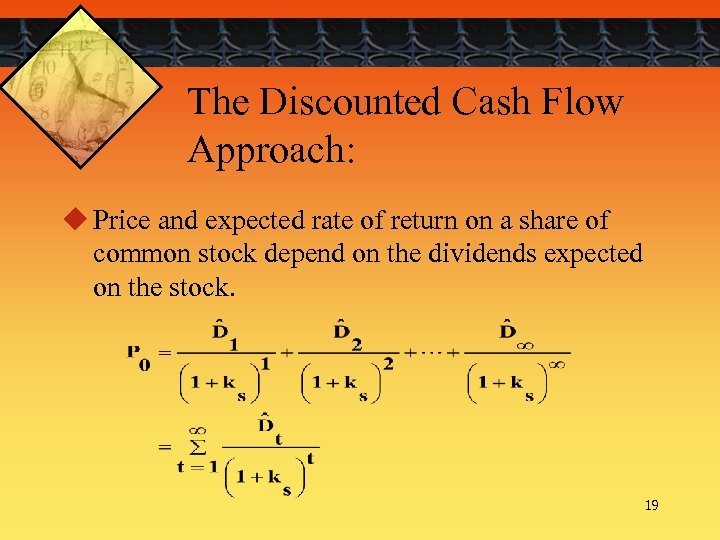The Discounted Cash Flow Approach: u Price and expected rate of return on a share of common stock depend on the dividends expected on the stock. 19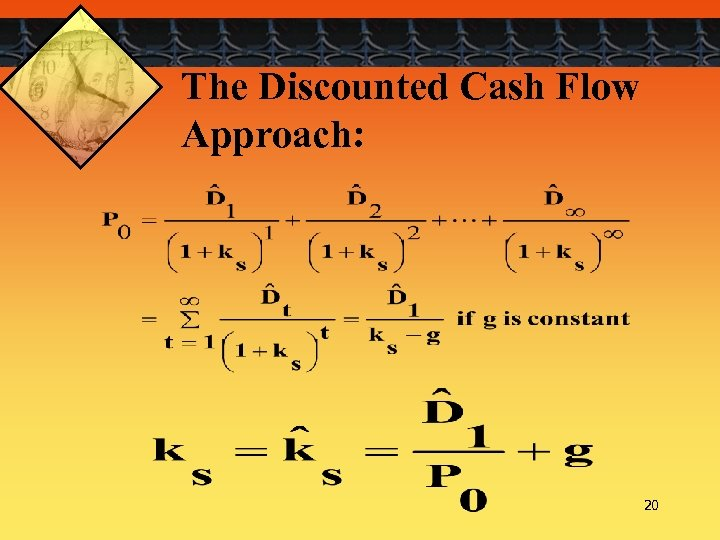The Discounted Cash Flow Approach: 20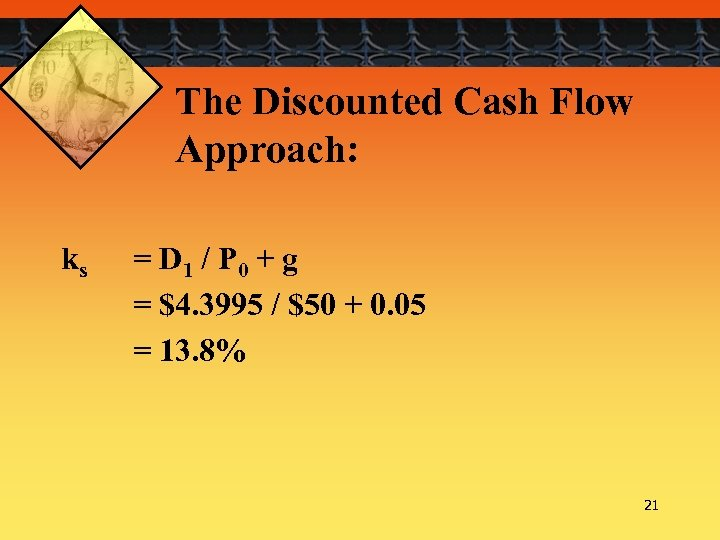The Discounted Cash Flow Approach: ks = D 1 / P 0 + g = \$4. 3995 / \$50 + 0. 05 = 13. 8% 21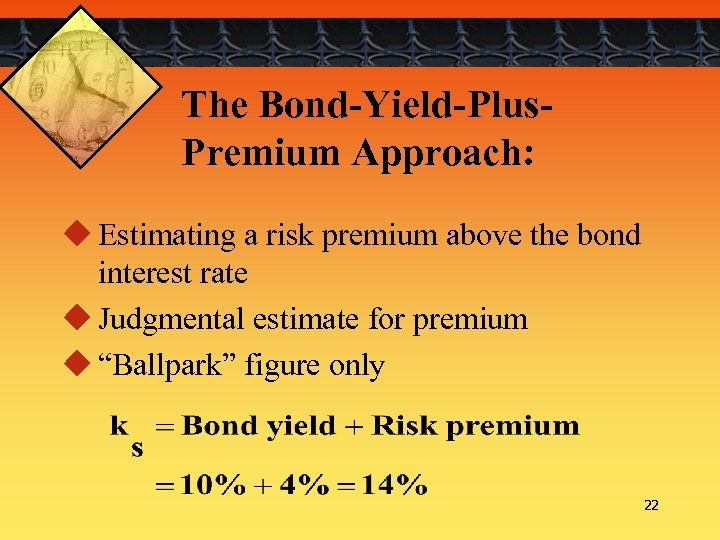The Bond-Yield-Plus. Premium Approach: u Estimating a risk premium above the bond interest rate u Judgmental estimate for premium u “Ballpark” figure only 22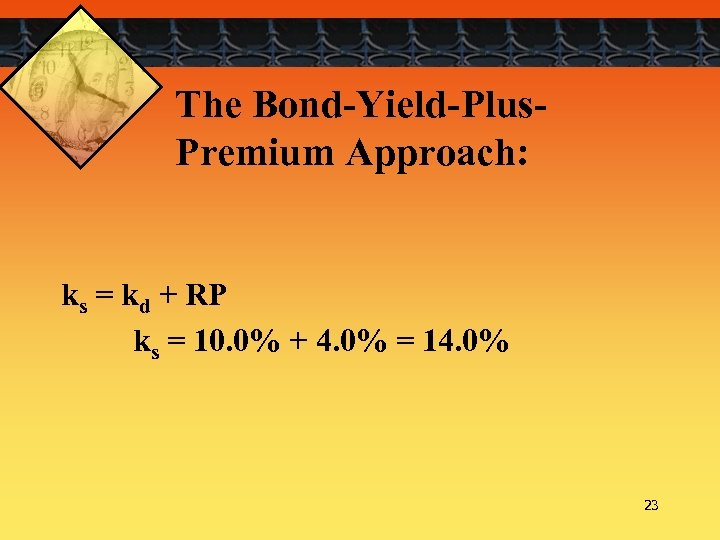The Bond-Yield-Plus. Premium Approach: ks = kd + RP ks = 10. 0% + 4. 0% = 14. 0% 23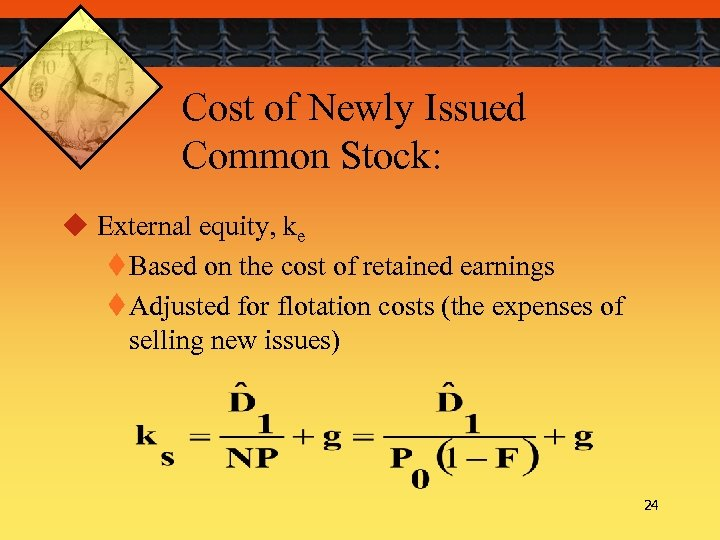Cost of Newly Issued Common Stock: u External equity, ke t Based on the cost of retained earnings t Adjusted for flotation costs (the expenses of selling new issues) 24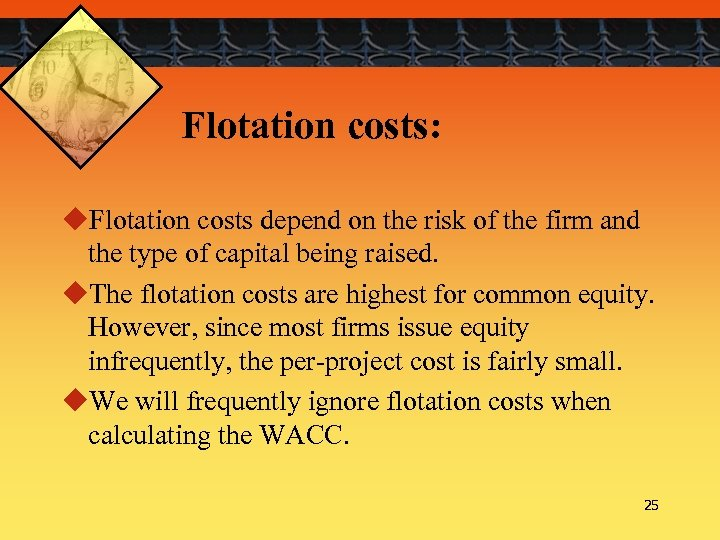Flotation costs: u. Flotation costs depend on the risk of the firm and the type of capital being raised. u. The flotation costs are highest for common equity. However, since most firms issue equity infrequently, the per-project cost is fairly small. u. We will frequently ignore flotation costs when calculating the WACC. 25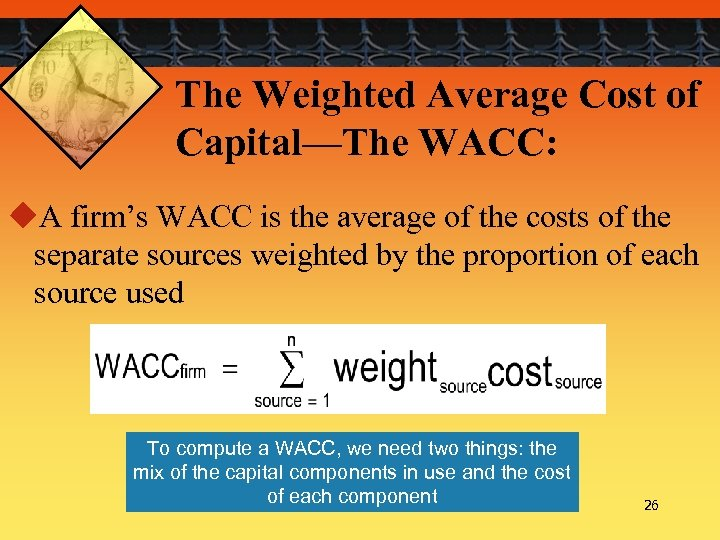The Weighted Average Cost of Capital—The WACC: u. A firm’s WACC is the average of the costs of the separate sources weighted by the proportion of each source used To compute a WACC, we need two things: the mix of the capital components in use and the cost of each component 26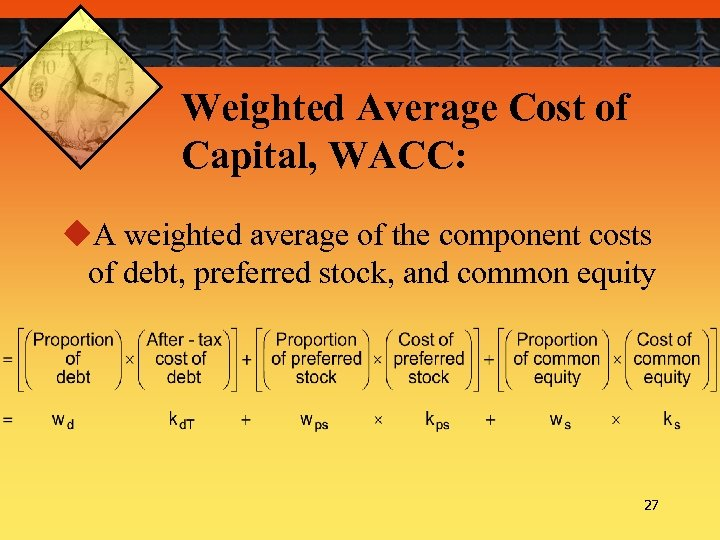Weighted Average Cost of Capital, WACC: u. A weighted average of the component costs of debt, preferred stock, and common equity 27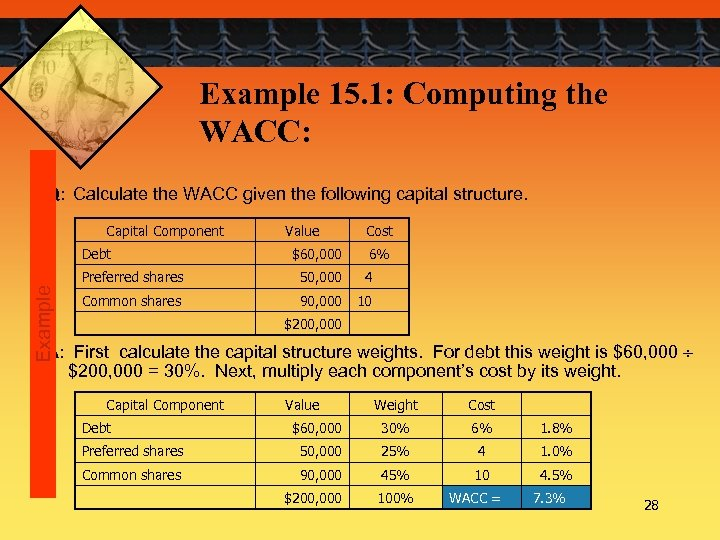Example 15. 1: Computing the WACC: Q: Calculate the WACC given the following capital structure. Capital Component Example Debt Value \$60, 000 Cost 6% Preferred shares 50, 000 4 Common shares 90, 000 10 \$200, 000 A: First calculate the capital structure weights. For debt this weight is \$60, 000 \$200, 000 = 30%. Next, multiply each component’s cost by its weight. Capital Component Weight Cost \$60, 000 30% 6% 1. 8% Preferred shares 50, 000 25% 4 1. 0% Common shares 90, 000 45% 10 4. 5% \$200, 000 100% Debt Value WACC = 7. 3% 2829